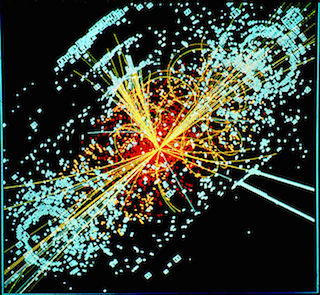# Super-Collider

Probability Level 3At time $t = 0$ a particle is at point $0$ on the real line. At time $t=1$, the particle divides into two and instantaneously after division, one particle moves one unit to the left and the other moves one unit to the right. At time $t = 2$, each of these particles gets divided into $2$ and one of the two new particles move to the right by 1 unit and the other moves to the left by 1 unit. Whenever two particles meet on the real line they annihilate (destroy) each other completely leaving not a single trace behind.

How many particles will be there at time $t = 8193$?

×